• Размер: 2.4 Mегабайта
• Количество слайдов: 27

## Описание презентации Project Analysis and Evaluation G. Kholjigitov FIN-311 — по слайдам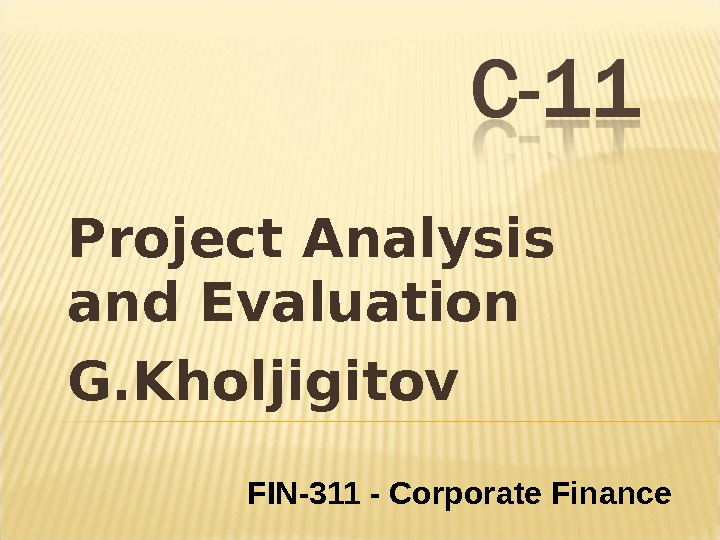Project Analysis and Evaluation G. Kholjigitov FIN-311 — Corporate Finance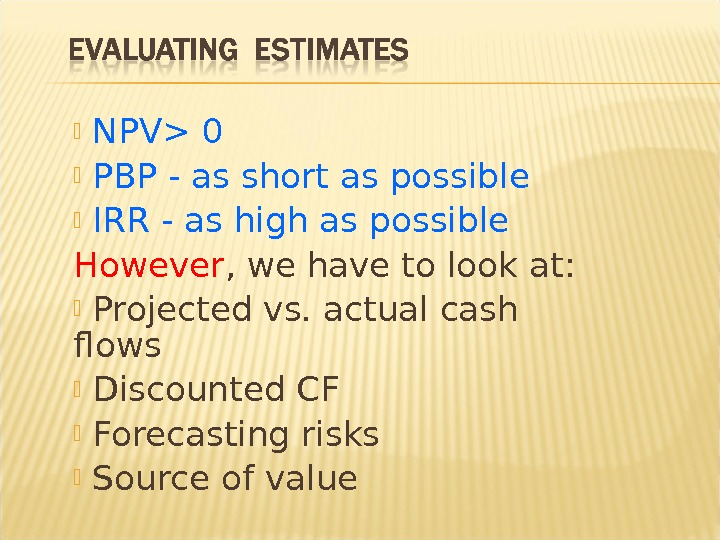NPV> 0 PBP — as short as possible IRR — as high as possible However , we have to look at: Projected vs. actual cash flows Discounted CF Forecasting risks Source of value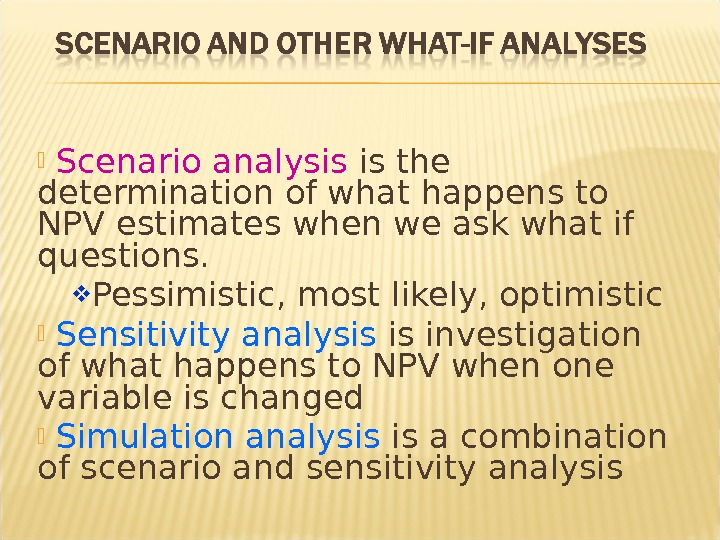Scenario analysis is the determination of what happens to NPV estimates when we ask what if questions. Pessimistic, most likely, optimistic Sensitivity analysis is investigation of what happens to NPV when one variable is changed Simulation analysis is a combination of scenario and sensitivity analysis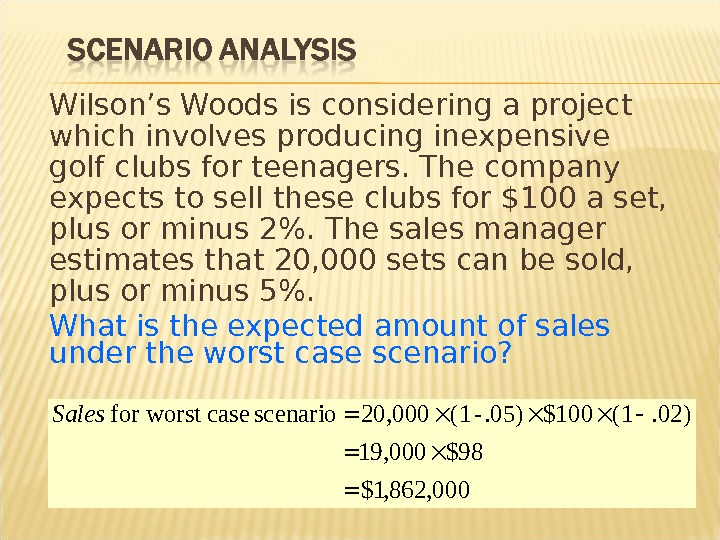Wilson’s Woods is considering a project which involves producing inexpensive golf clubs for teenagers. The company expects to sell these clubs for \$100 a set, plus or minus 2%. The sales manager estimates that 20, 000 sets can be sold, plus or minus 5%. What is the expected amount of sales under the worst case scenario? 000, 862, 1\$ 98\$19, 000 . 02)(1\$100. 05)- (1 20, 000 scenario casefor worst Sales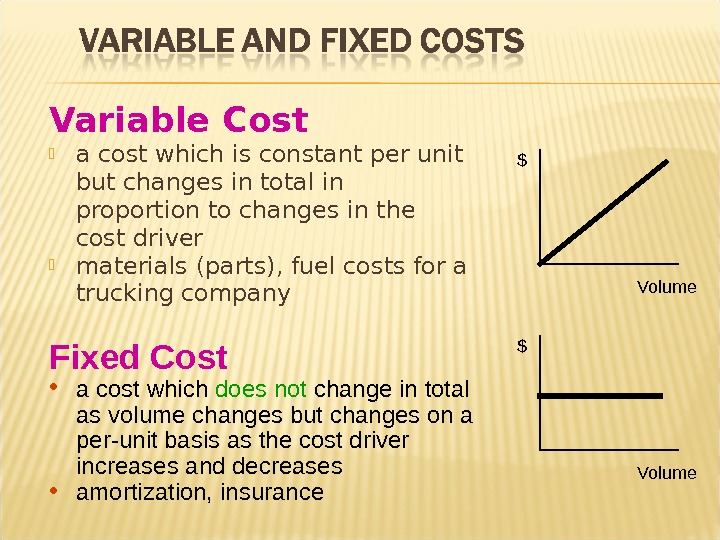Variable Cost a cost which is constant per unit but changes in total in proportion to changes in the cost driver materials (parts), fuel costs for a trucking company \$ Volume. Fixed Cost • a cost which does not change in total as volume changes but changes on a per-unit basis as the cost driver increases and decreases • amortization, insurance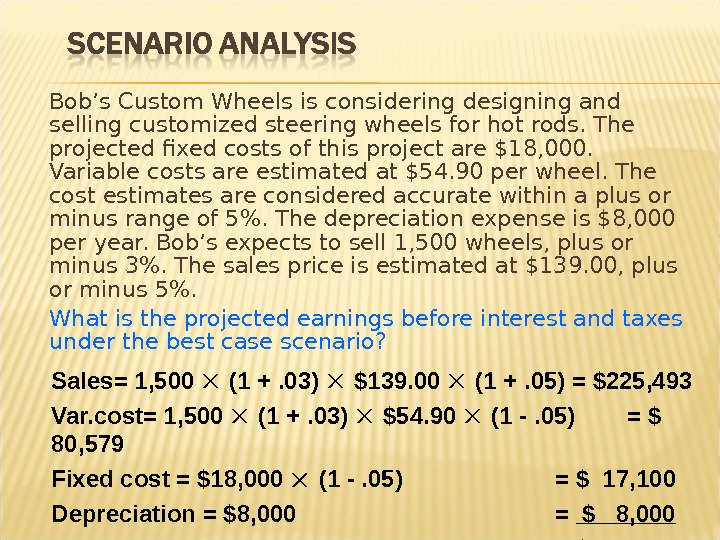Bob’s Custom Wheels is considering designing and selling customized steering wheels for hot rods. The projected fixed costs of this project are \$18, 000. Variable costs are estimated at \$54. 90 per wheel. The cost estimates are considered accurate within a plus or minus range of 5%. The depreciation expense is \$8, 000 per year. Bob’s expects to sell 1, 500 wheels, plus or minus 3%. The sales price is estimated at \$139. 00, plus or minus 5%. What is the projected earnings before interest and taxes under the best case scenario? Sales= 1, 500 (1 +. 03) \$139. 00 (1 +. 05) = \$225, 493 Var. cost= 1, 500 (1 +. 03) \$54. 90 (1 -. 05) = \$ 80, 579 Fixed cost = \$18, 000 (1 -. 05) = \$ 17, 100 Depreciation = \$8, 000 = \$ 8, 000 EBIT = \$119,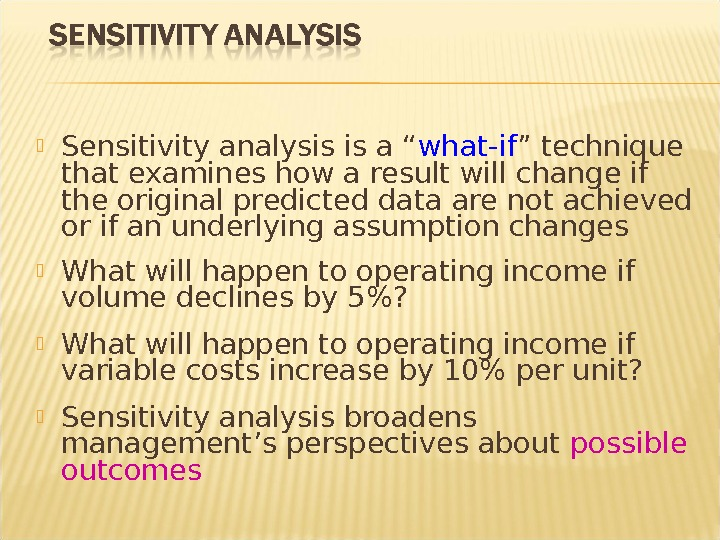Sensitivity analysis is a “ what-if ” technique that examines how a result will change if the original predicted data are not achieved or if an underlying assumption changes What will happen to operating income if volume declines by 5%? What will happen to operating income if variable costs increase by 10% per unit? Sensitivity analysis broadens management’s perspectives about possible outcomes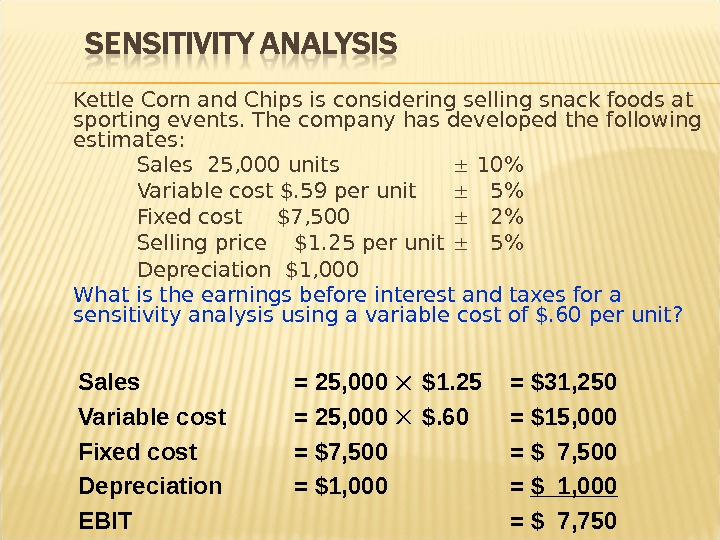Kettle Corn and Chips is considering selling snack foods at sporting events. The company has developed the following estimates: Sales 25, 000 units 10% Variable cost \$. 59 per unit 5% Fixed cost \$7, 500 2% Selling price \$1. 25 per unit 5% Depreciation \$1, 000 What is the earnings before interest and taxes for a sensitivity analysis using a variable cost of \$. 60 per unit? Sales = 25, 000 \$1. 25 = \$31, 250 Variable cost = 25, 000 \$. 60 = \$15, 000 Fixed cost = \$7, 500 = \$ 7, 500 Depreciation = \$1, 000 = \$ 1, 000 EBIT = \$ 7,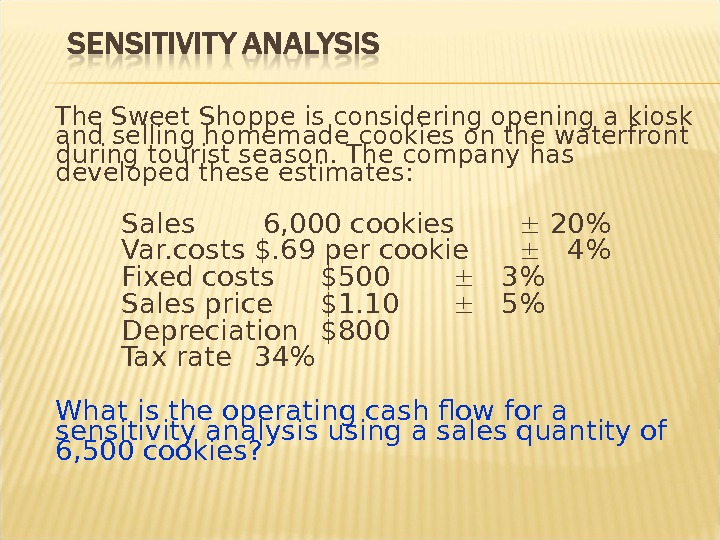The Sweet Shoppe is considering opening a kiosk and selling homemade cookies on the waterfront during tourist season. The company has developed these estimates: Sales 6, 000 cookies 20% Var. costs \$. 69 per cookie 4% Fixed costs \$500 3% Sales price \$1. 10 5% Depreciation \$800 Tax rate 34% What is the operating cash flow for a sensitivity analysis using a sales quantity of 6, 500 cookies?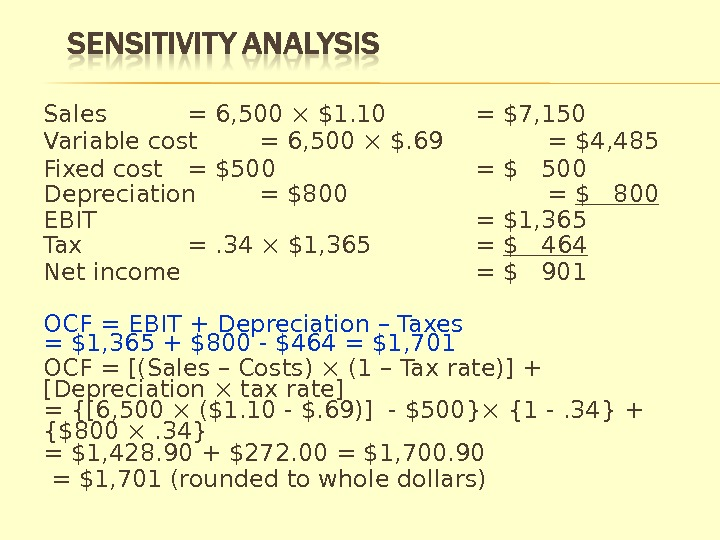Sales = 6, 500 \$1. 10 = \$7, 150 Variable cost = 6, 500 \$. 69 = \$4, 485 Fixed cost = \$500 = \$ 500 Depreciation = \$800 = \$ 800 EBIT = \$1, 365 Tax =. 34 \$1, 365 = \$ 464 Net income = \$ 901 OCF = EBIT + Depreciation – Taxes = \$1, 365 + \$800 — \$464 = \$1, 701 OCF = [(Sales – Costs) (1 – Tax rate)] + [Depreciation tax rate] = {[6, 500 (\$1. 10 — \$. 69)] — \$500} {1 -. 34} + {\$800 . 34} = \$1, 428. 90 + \$272. 00 = \$1, 700. 90 = \$1, 701 (rounded to whole dollars)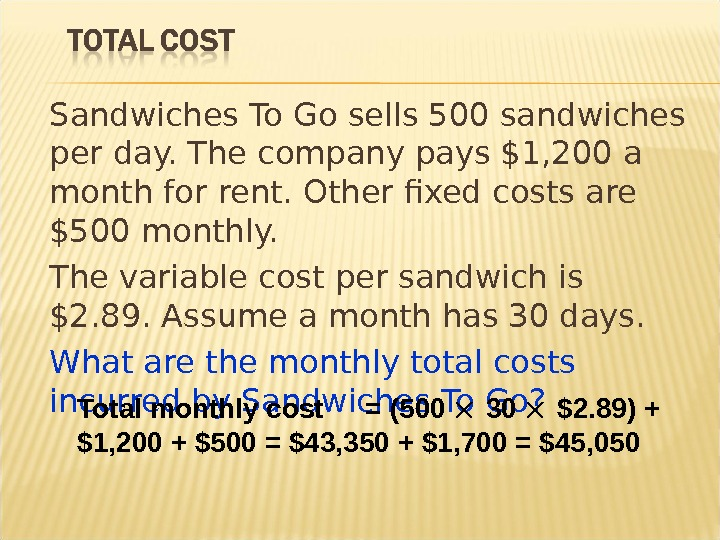Sandwiches To Go sells 500 sandwiches per day. The company pays \$1, 200 a month for rent. Other fixed costs are \$500 monthly. The variable cost per sandwich is \$2. 89. Assume a month has 30 days. What are the monthly total costs incurred by Sandwiches To Go? Total monthly cost = (500 30 \$2. 89) + \$1, 200 + \$500 = \$43, 350 + \$1, 700 = \$45,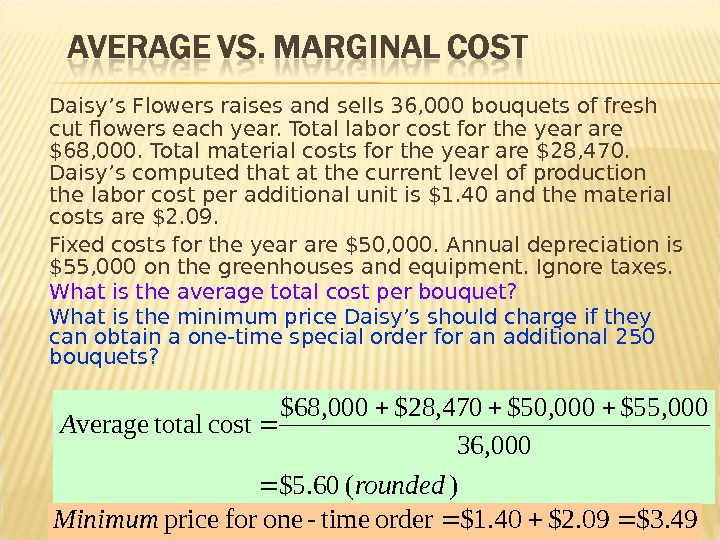Daisy’s Flowers raises and sells 36, 000 bouquets of fresh cut flowers each year. Total labor cost for the year are \$68, 000. Total material costs for the year are \$28, 470. Daisy’s computed that at the current level of production the labor cost per additional unit is \$1. 40 and the material costs are \$2. 09. Fixed costs for the year are \$50, 000. Annual depreciation is \$55, 000 on the greenhouses and equipment. Ignore taxes. What is the average total cost per bouquet? What is the minimum price Daisy’s should charge if they can obtain a one-time special order for an additional 250 bouquets? )( 60. 5\$ 36, 000 \$55, 000 \$50, 000\$28, 470 \$68, 000 cost totalverage rounded. A \$3. 49 \$2. 09 \$1. 40 order time-onefor price Minimum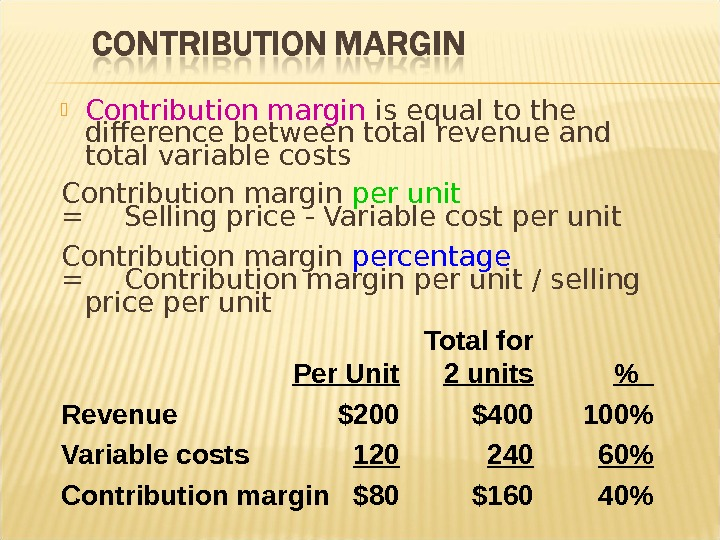Contribution margin is equal to the difference between total revenue and total variable costs Contribution margin per unit = Selling price — Variable cost per unit Contribution margin percentage = Contribution margin per unit / selling price per unit Total for Per Unit 2 units % Revenue \$200 \$400 100% Variable costs 120 240 60% Contribution margin \$80 \$160 40%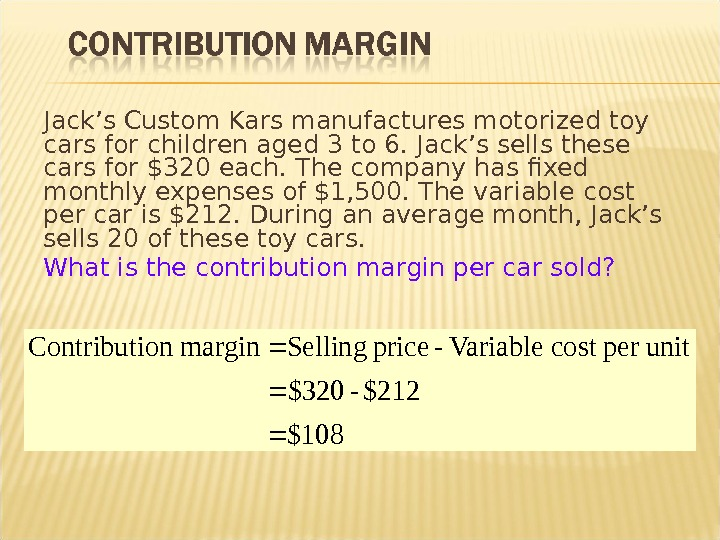Jack’s Custom Kars manufactures motorized toy cars for children aged 3 to 6. Jack’s sells these cars for \$320 each. The company has fixed monthly expenses of \$1, 500. The variable cost per car is \$212. During an average month, Jack’s sells 20 of these toy cars. What is the contribution margin per car sold? \$108 \$212 — \$320 unitper cost Variable — price Selling margin on Contributi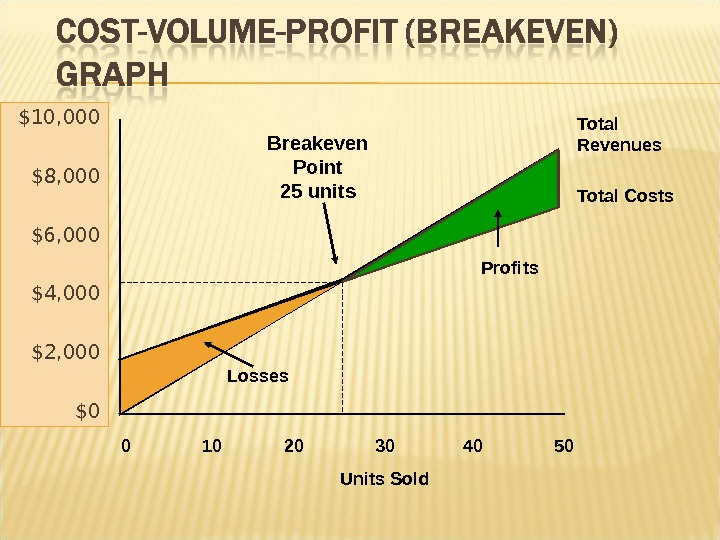\$10, 000 \$8, 000 \$6, 000 \$4, 000 \$2, 000 \$0 0 10 20 30 40 50 Units Sold Total Revenues Total Costs. Breakeven Point 25 units Profits Losses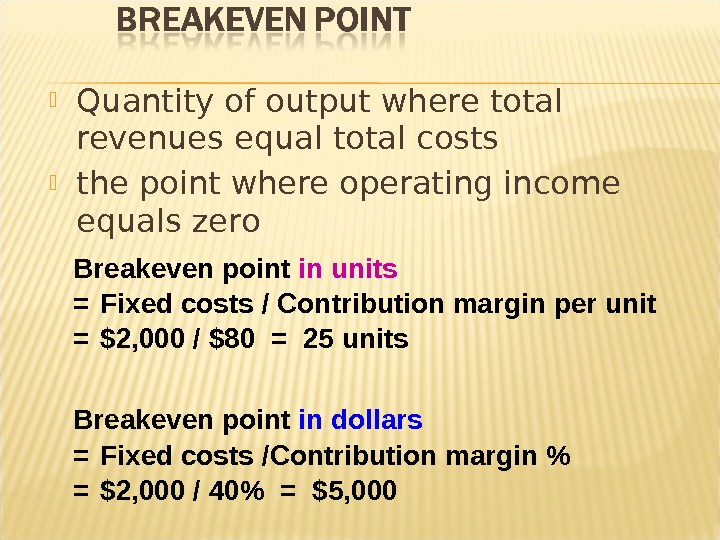Quantity of output where total revenues equal total costs the point where operating income equals zero Breakeven point in units = Fixed costs / Contribution margin per unit = \$2, 000 / \$80 = 25 units Breakeven point in dollars = Fixed costs /Contribution margin % = \$2, 000 / 40% = \$5,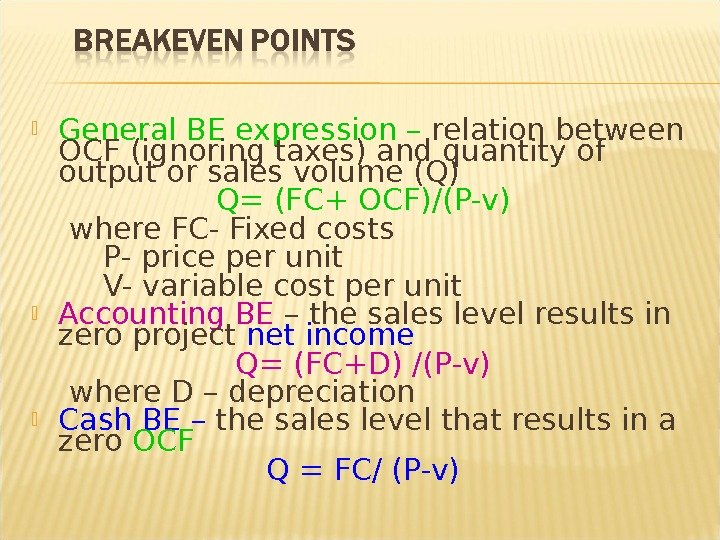General BE expression – relation between OCF (ignoring taxes) and quantity of output or sales volume (Q) Q= (FC+ OCF)/(P-v) where FC- Fixed costs P- price per unit V- variable cost per unit Accounting BE – the sales level results in zero project net income Q= (FC+D) /(P-v) where D – depreciation Cash BE – the sales level that results in a zero OCF Q = FC/ (P-v)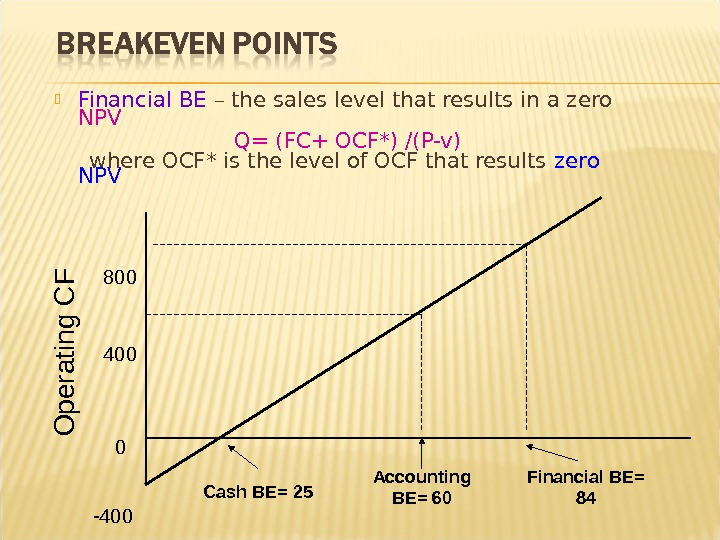Financial BE – the sales level that results in a zero NPV Q= (FC+ OCF*) /(P-v) where OCF* is the level of OCF that results zero NPV Financial BE= 84 Cash BE= 25 Accounting BE= 60 O p e ra tin g C F —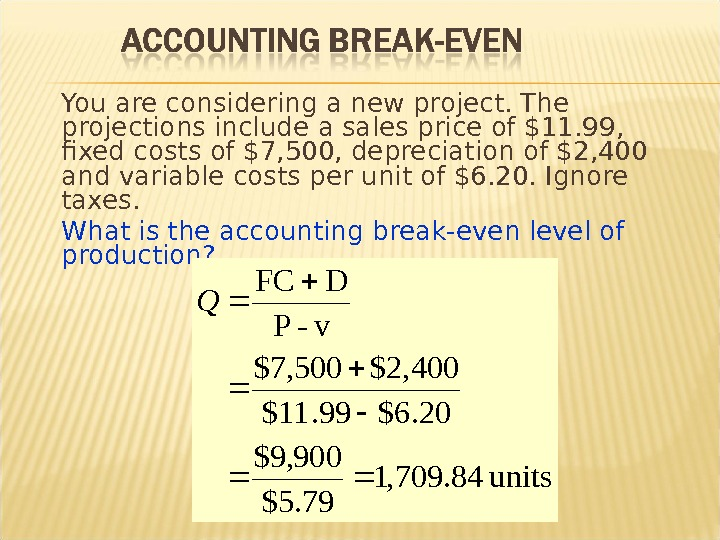You are considering a new project. The projections include a sales price of \$11. 99, fixed costs of \$7, 500, depreciation of \$2, 400 and variable costs per unit of \$6. 20. Ignore taxes. What is the accounting break-even level of production? units 84. 709, 1 79. 5\$ 900, 9\$ 20. 6\$99. 11\$ 400, 2\$500, 7\$ v- P D FC Q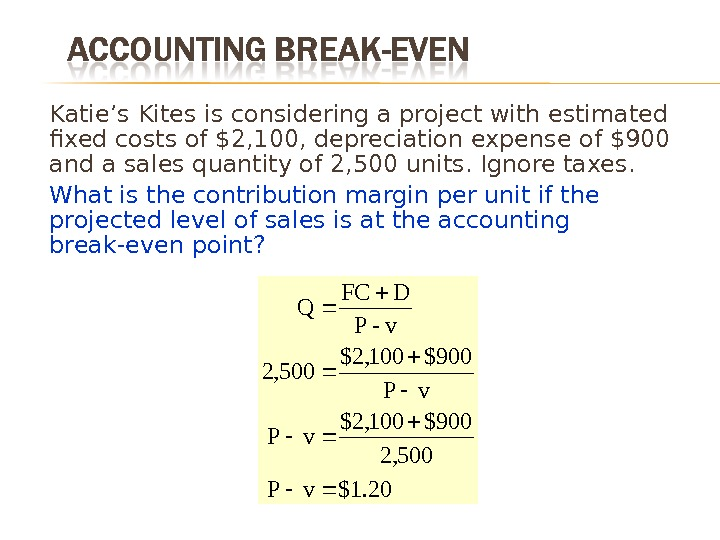Katie’s Kites is considering a project with estimated fixed costs of \$2, 100, depreciation expense of \$900 and a sales quantity of 2, 500 units. Ignore taxes. What is the contribution margin per unit if the projected level of sales is at the accounting break-even point? 20. 1\$v. P 500, 2 900\$100, 2\$ v. P 900\$100, 2\$ 500, 2 v- P D FC Q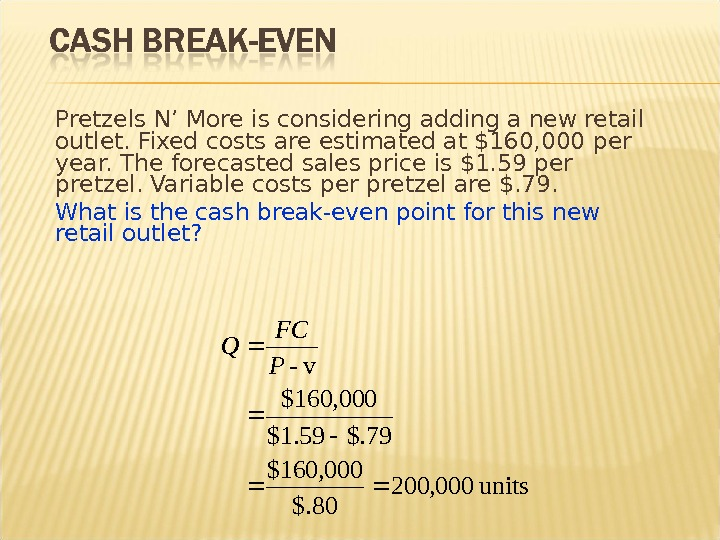Pretzels N’ More is considering adding a new retail outlet. Fixed costs are estimated at \$160, 000 per year. The forecasted sales price is \$1. 59 per pretzel. Variable costs per pretzel are \$. 79. What is the cash break-even point for this new retail outlet? units 000, 200 80\$. 000, 160\$ 79\$. 59. 1\$ 000, 160\$ v- P FC Q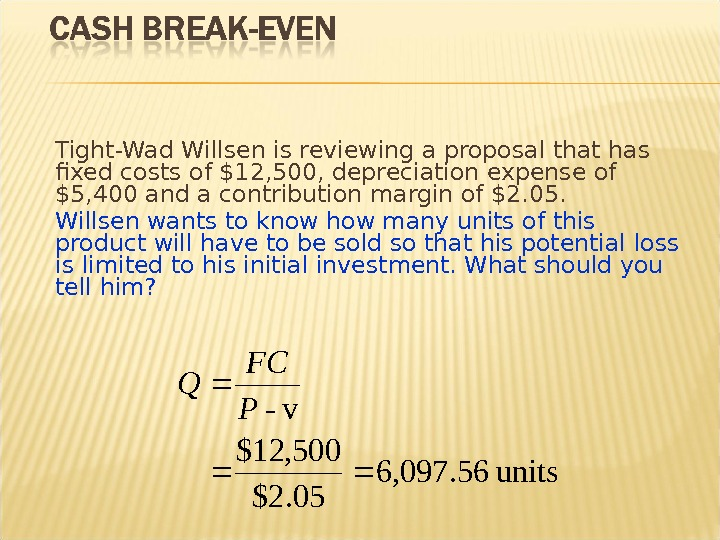Tight-Wad Willsen is reviewing a proposal that has fixed costs of \$12, 500, depreciation expense of \$5, 400 and a contribution margin of \$2. 05. Willsen wants to know how many units of this product will have to be sold so that his potential loss is limited to his initial investment. What should you tell him? units 56. 097, 6 05. 2\$ 500, 12\$ v- P FC Q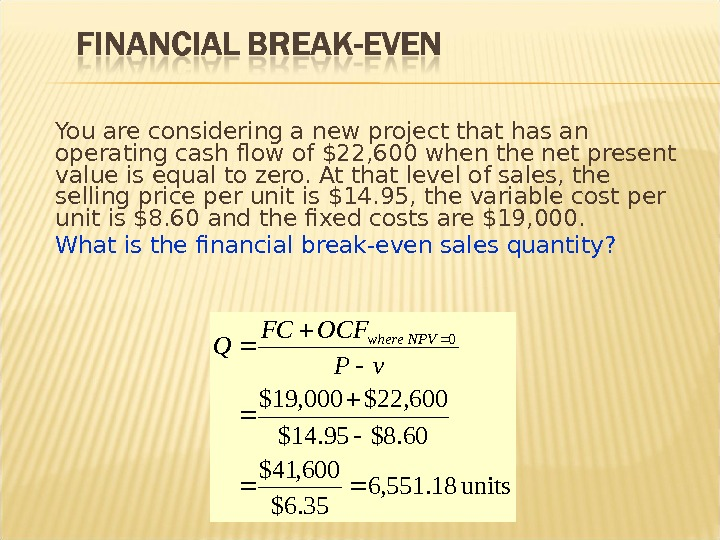You are considering a new project that has an operating cash flow of \$22, 600 when the net present value is equal to zero. At that level of sales, the selling price per unit is \$14. 95, the variable cost per unit is \$8. 60 and the fixed costs are \$19, 000. What is the financial break-even sales quantity? units 18. 551, 6 35. 6\$ 600, 41\$ 60. 8\$95. 14\$ 600, 22\$000, 19\$ 0 v. POCFFC Q NPVwhere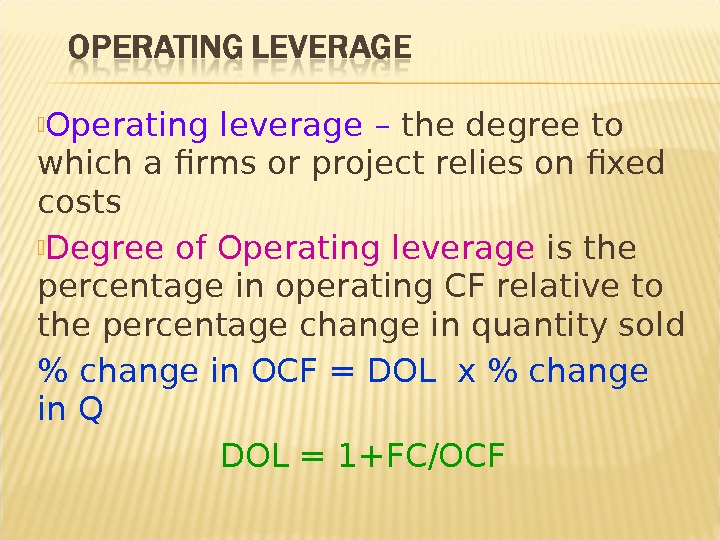Operating leverage – the degree to which a firms or project relies on fixed costs Degree of Operating leverage is the percentage in operating CF relative to the percentage change in quantity sold % change in OCF = DOL x % change in Q DOL = 1+FC/O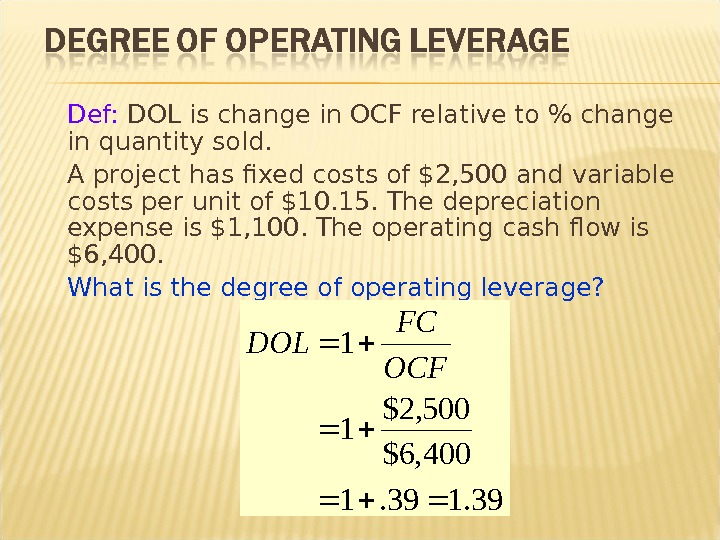Def: DOL is change in OCF relative to % change in quantity sold. A project has fixed costs of \$2, 500 and variable costs per unit of \$10. 15. The depreciation expense is \$1, 100. The operating cash flow is \$6, 400. What is the degree of operating leverage? 39. 1 400, 6\$ 500, 2\$ 11 OCF FC DOL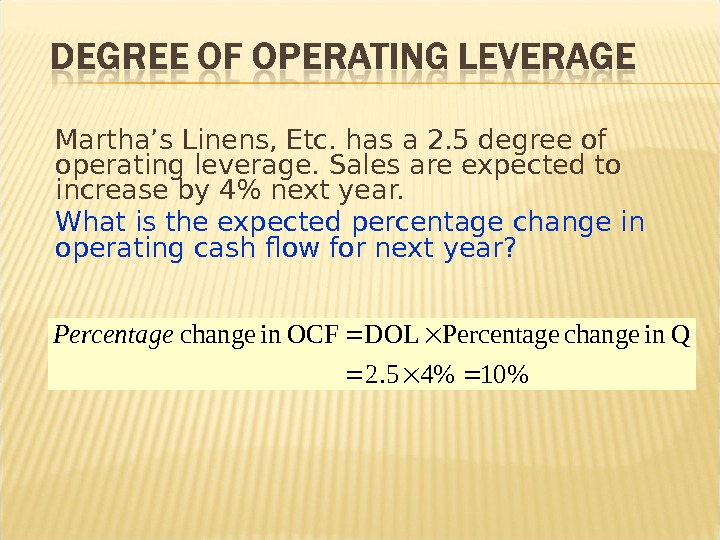Martha’s Linens, Etc. has a 2. 5 degree of operating leverage. Sales are expected to increase by 4% next year. What is the expected percentage change in operating cash flow for next year? %10%45. 2 Qin change Percentage DOL OCFin change Percentage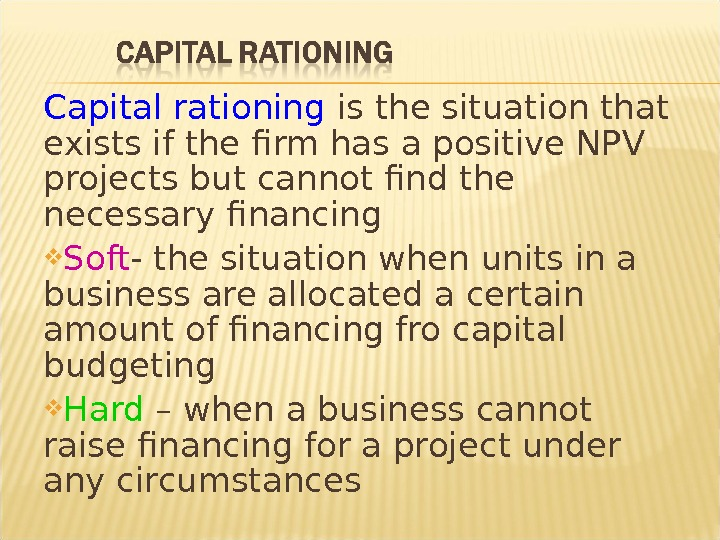Capital rationing is the situation that exists if the firm has a positive NPV projects but cannot find the necessary financing Soft — the situation when units in a business are allocated a certain amount of financing fro capital budgeting Hard – when a business cannot raise financing for a project under any circumstances